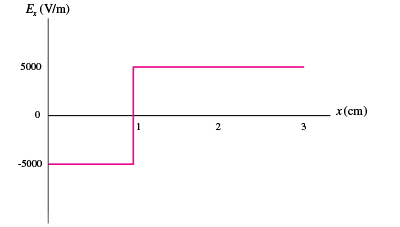# Problem: Select the correct graph of Ex versus x.  a.b.c.  d.

###### FREE Expert Solution

Electric field:

$\overline{){\mathbf{E}}{\mathbf{=}}{\mathbf{-}}\frac{\mathbf{V}}{\mathbf{d}}}$

This implies that the electric field is the negative gradient of the potential.

The value of the electric field can be determined if the slope is known for the plot of Potential (V) vs. Distance (x).###### Problem Details

Select the correct graph of Ex versus x.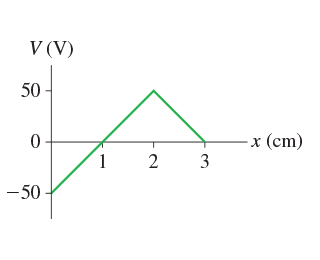a.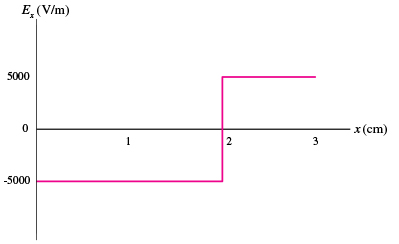b.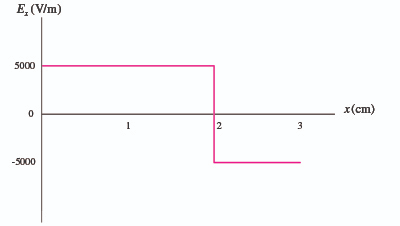c.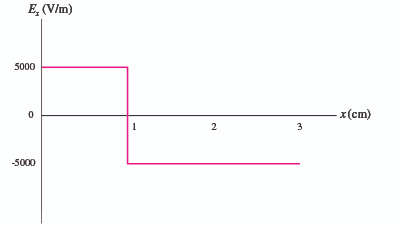d.